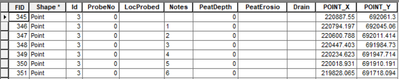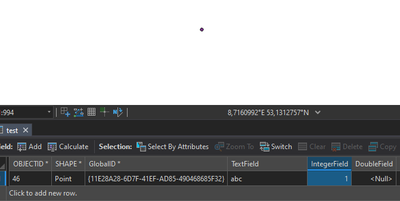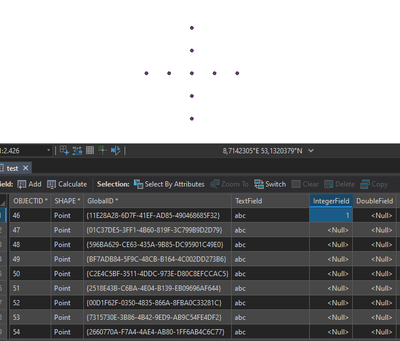# I have 70 points which need additional points added at 25 and 50m N, S, E, W

408
3
03-15-2022 04:10 AM
Labels (3)New Contributor II

Hello - this is a new task for me so please answer as if I'm in primary school. Shapefile contains 70 points which each need a point added at 25 and 50 m in each direction. What is the easiest way to do/automate this? So far I have only added x, y fields.  Thanks.Tags (3)
1 Solution

Accepted SolutionsbyMVP Regular Contributor

Easiest way is probably to use Python:

• Open the Python window
• Copy and paste the script below
• Modify the layer and fields variables
• Run
• You may have to refresh the map to see the changes
``````# Name of the layer (if loaded in map) or path to the feature class / shape file
layer = "test"
# fields that are copied. First one has to be SHAPE@
fields = ["SHAPE@", "TextField"]

original_points = [row for row in arcpy.da.SearchCursor(layer, fields)]

with arcpy.da.InsertCursor(layer, fields) as cursor:
for point in original_points:
# create the 8 new coordinate pairs
coords = point.firstPoint
new_coords = [
[coords.X - 25, coords.Y],
[coords.X - 50, coords.Y],
[coords.X + 25, coords.Y],
[coords.X + 50, coords.Y],
[coords.X, coords.Y - 25],
[coords.X, coords.Y - 50],
[coords.X, coords.Y + 25],
[coords.X, coords.Y + 50],
]

for nc in new_coords:
# create a copy of the original point's attributes
new_point = list(point)
# change the copy's geometry
new_point = arcpy.PointGeometry(arcpy.Point(nc, nc))
# write copied point to shape file
cursor.insertRow(new_point)``````

BeforeAfter (note that I only specified "TextField" to be copied)Have a great day!
Johannes
3 RepliesbyMVP Regular Contributor

Easiest way is probably to use Python:

• Open the Python window
• Copy and paste the script below
• Modify the layer and fields variables
• Run
• You may have to refresh the map to see the changes
``````# Name of the layer (if loaded in map) or path to the feature class / shape file
layer = "test"
# fields that are copied. First one has to be SHAPE@
fields = ["SHAPE@", "TextField"]

original_points = [row for row in arcpy.da.SearchCursor(layer, fields)]

with arcpy.da.InsertCursor(layer, fields) as cursor:
for point in original_points:
# create the 8 new coordinate pairs
coords = point.firstPoint
new_coords = [
[coords.X - 25, coords.Y],
[coords.X - 50, coords.Y],
[coords.X + 25, coords.Y],
[coords.X + 50, coords.Y],
[coords.X, coords.Y - 25],
[coords.X, coords.Y - 50],
[coords.X, coords.Y + 25],
[coords.X, coords.Y + 50],
]

for nc in new_coords:
# create a copy of the original point's attributes
new_point = list(point)
# change the copy's geometry
new_point = arcpy.PointGeometry(arcpy.Point(nc, nc))
# write copied point to shape file
cursor.insertRow(new_point)``````

BeforeAfter (note that I only specified "TextField" to be copied)Have a great day!
JohannesNew Contributor II

Thank you Johannes, I will try this.New Contributor II

@JohannesLindner  this was a brilliant solution. It took me a few times to play with it to get the fields right, but in the end it worked and your solution really impressed my team. A few made comments they wouldn't have thought of doing it that way, Very much appreciated. Apologies for taking a week to respond.